Return to edHelper.com January Theme Units Classroom Helpers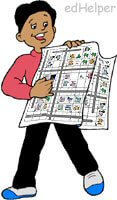Community MapsTheme Unit

Map Key - List of Symbols
Use the map key printable as a reference for use with all of the other printables:
Map Key Printable

Easier Maps (larger drawings, smaller community)
With grid lines
Symbols
Fill in the blanks with north, south, east, west
Grid Coordinates: Circle the building or object located at the grid coordinates
Grid Coordinates: Write the grid coordinates of the buildings
Write directions
Calculate distance true/false questions (feet)
Calculate distance true/false questions (meters)
Calculate distance (feet)
Calculate distance (meters)

No grid lines
Symbols
Fill in the blanks with north, south, east, west
Grid Coordinates: Circle the building or object located at the grid coordinates
Grid Coordinates: Write the grid coordinates of the buildings
Write directions
Calculate distance true/false questions (feet)
Calculate distance true/false questions (meters)
Calculate distance (feet)
Calculate distance (meters)

Maps (smaller graphics; grid is 10 x 10)
With grid lines
Symbols
Fill in the blanks with north, south, east, west
Grid Coordinates: Circle the building or object located at the grid coordinates
Grid Coordinates: Write the grid coordinates of the buildings
Write directions
Calculate distance true/false questions (feet)
Calculate distance true/false questions (meters)
Calculate distance (feet)
Calculate distance (meters)

No grid lines
Symbols
Fill in the blanks with north, south, east, west
Grid Coordinates: Circle the building or object located at the grid coordinates
Grid Coordinates: Write the grid coordinates of the buildings
Write directions
Calculate distance true/false questions (feet)
Calculate distance true/false questions (meters)
Calculate distance (feet)
Calculate distance (meters)

Book Units

More Difficult Maps (grid is 13 x 13)
With grid lines
Symbols
Fill in the blanks with north, south, east, west
Grid Coordinates: Circle the building or object located at the grid coordinates
Grid Coordinates: Write the grid coordinates of the buildings
Write directions
Calculate distance true/false questions (feet)
Calculate distance true/false questions (meters)
Calculate distance (feet)
Calculate distance (meters)

No grid lines
Symbols
Fill in the blanks with north, south, east, west
Grid Coordinates: Circle the building or object located at the grid coordinates
Grid Coordinates: Write the grid coordinates of the buildings
Write directions
Calculate distance true/false questions (feet)
Calculate distance true/false questions (meters)
Calculate distance (feet)
Calculate distance (meters)

Books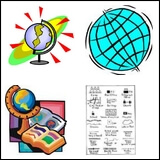Mapping ABC Style (Grades 3-6)

Writing

Document Based Activities

Glyphs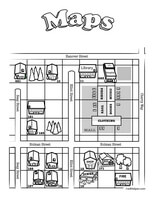Maps Glyph

Analogies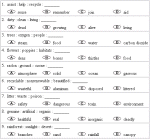Community Map Analogies

More Activities and Printables
 Communities

Following Directions and Symbols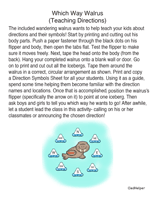Which Way Walrus (Teaching Directions)

Map Activities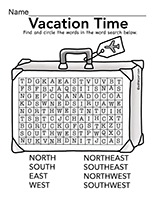Map Activities

Teacher Recommendations:  Worksheet and Lesson Plan Activity Ideas

50 States

Math

Money

Calendar

Word Search

Poetry

Weather

Shapes

Science

Solar System

Back To School

Cause And Effect

More Activities, Lesson Plans, and Worksheets

Have a suggestion or would like to leave feedback?